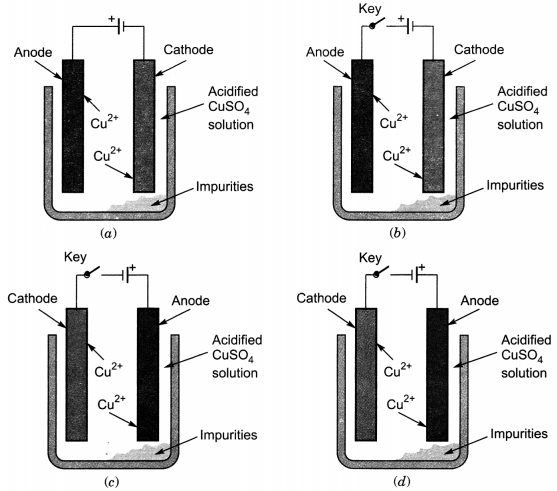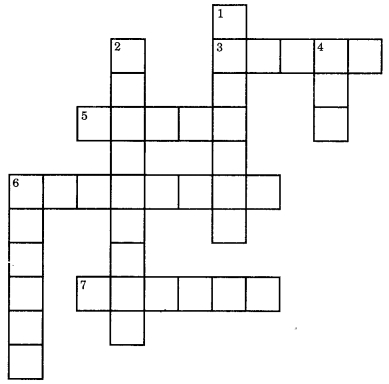# MCQ Questions for Class 10 Science Chapter 3 Metals and Non-metals with Answers

Check the below NCERT MCQ Questions for Class 10 Science Chapter 3 Metals and Non-metals with Answers Pdf free download. MCQ Questions for Class 10 Science with Answers were prepared based on the latest exam pattern. We have Provided Metals and Non-metals Class 10 Science MCQs Questions with Answers to help students understand the concept very well. https://mcqquestions.guru/mcq-questions-for-class-10-science-chapter-3/

You can refer to NCERT Solutions for Class 10 Science Chapter 3 Metals and Non-metals to revise the concepts in the syllabus effectively and improve your chances of securing high marks in your board exams.

## Metals and Non-metals Class 10 MCQs Questions with Answers

Metals And Non Metals Class 10 MCQ Question 1.
The ability of metals to be drawn into thin wires is known as
(a) ductility
(b) malleability
(c) sonority
(d) conductivity

Metal And Non Metals Class 10 MCQ Question 2.
Aluminium is used for making cooking utensils. Which of the following properties of aluminium are responsible for the same?
(i) Good thermal conductivity
(ii) Good electrical conductivity
(iii) Ductility
(iv) High melting point
(a) (i) and (ii)
(b) (i) and (iii)
(c) (ii) and (iii)
(d) (i) and (iv)

Metals And Nonmetals Class 10 MCQ Question 3.
Due to its semiconductor properties the non-metal used in computer, T.V. etc. is
(a) Carbon
(b) Silicon
(c) Bromine
(d) Fluorine

Class 10 Metals And Non Metals MCQ Question 4.
What happens when calcium is treated with water?
(i) It does not react with water.
(ii) It reacts violently with water.
(iii) It reacts less violently with water.
(iv) Bubbles of hydrogen gas formed stick to the surface of calcium.
(a) (i) and (iv)
(b) (ii) and (iii)
(c) (i) and (ii)
(d) (iii) and (iv)

Class 10 Science Chapter 3 MCQ Question 5.
Generally metals react with acids to give salt and hydrogen gas. Which of the following acids does not give hydrogen gas on reacting with metals (except Mn and Mg)?
(a) H2SO4
(b) HCl
(c) HNO3
(d) All of these

Metals And Non Metals MCQ Question 6.
Which of the following metals are obtained by electrolysis of their chlorides in molten state?
(i) Na
(ii) Ca
(iii) Fe
(iv) Cu
(a) (i) and (iv)
(b) (iii) and (iv)
(c) (i) and (iii)
(d) (i) and (ii)

Ch 3 Science Class 10 MCQ Question 7.
An alloy reacted with dilute hydrochloric acid to produce a gas which ‘pops’ a lighted splint. The residue reacted with dilute nitric acid to form a blue solution. Which one of the following pairs of metals is present in the alloy?
(c) Copper and magnesium

Class 10 Science Ch 3 MCQ Question 8.
Which of the following metals exist in their native state in nature?
(i) Cu
(ii) Au
(iii) Zn
(iv) Ag
(a) (i) and (ii)
(b) (ii) and (iii)
(c) (ii) and (iv)
(d) (iii) and (iv)

MCQ On Metals And Non Metals Class 10 Question 9.
Metals are refined by using different methods. Which of the following metals are refined by electrolytic refining?
(i) Au
(ii) Cu
(iii) Na
(iv) K
(a) (i) and (ii)
(b) (i) and (iii)
(c) (ii) and (iii)
(d) (iii)and (iv)

Metal And Non Metals MCQ Class 10 Question 10.
Metal M occurs in the Earth’s crust as its oxide M2O3. An alloy of this metal is used in making aircrafts. The ore of the metal M is
(a) magnetite
(b) haematite
(c) bauxite
(d) pyrolusite

MCQ On Metals And Non Metals Question 11.
Which one of the following four metals would be displaced from the solution of its salts by other three metals?
(a) Mg
(b) Ag
(c) Zn
(d) Cu

Metal And Non Metal Class 10 MCQ Question 12.
2 mL each of concentrated HCl, HNO3 and a mixture of concentrated HCl and concentrated HNO3 in the ratio of 3 : 1 were taken in test tubes labelled as A, B and C. A small piece of metal was put in each test tube. No change occurred in test tubes A and B but the metal got dissolved in test tube C respectively. The metal could be
(a) Al
(b) Au
(c) Cu
(d) Pt

MCQ Questions For Class 10 Science Chapter 3 Question 13.
Which one of the following figures correctly describes the process of electrolytic refining?MCQ Of Metal And Nonmetal Class 10 Question 14.
An element A is soft and can be cut with a knife. This is very reactive to air and cannot be kept in open. It reacts vigorously with water. Identify the element from the following:
(a) Mg
(b) Na
(c) P
(d) Ca

Chapter 3 Science Class 10 MCQ Question 15.
Alloys are homogeneous mixtures of a metal with a metal or non-metal. Which among the following alloys contain non-metal as one of its constituents?
(a) Brass
(b) Bronze
(c) Amalgam
(d) Steel

Question 16.
Reaction between X and Y, forms compound Z. X loses electron and Y gains electron. Which of the following properties is not shown by Z?
(a) Has high melting point
(b) Has low melting point
(c) Conducts electricity in molten state
(d) Occurs as solid

Answer: (b) Has low melting point

Question 17.
The electronic configurations of three elements X, Y and Z are X – 2, 8; Y – 2, 8, 7 and Z – 2, 8, 2. Which of the following is correct?
(a) X is a metal.
(b) Y is a metal.
(c) Z is a non-metal.
(d) Y is a non-metal and Z is a metal.

Answer: (d) Y is a non-metal and Z is a metal.

Question 18.
Generally, non-metals are not conductors of electricity. Which of the following is a good conductor of electricity?
(a) Diamond
(b) Graphite
(c) Sulphur
(d) Fullerene

Question 19.
Which of the following can undergo a chemical reaction?
(a) MgSO4 + Fe
(b) ZnSO2 + Fe
(c) MgSO2 + Pb
(d) CuSO2 + Fe

Question 20.
The atomic numbers of four elements A, B, C and D are 6, 8, 10 and 12 respectively. The two elements which can react to form ionic bonds (or ionic compound) are:
(a) A and D
(b) B and C
(c) A and C
(d) B and D

Question 21.
The atomic number of an element X is 19. The number of electrons in its ion X+ will be:
(a) 18
(b) 19
(c) 20
(d) 21

Question 22.
The atomic number of an element Y is 17. The number of electrons in its ion Y will be:
(a) 17
(b) 18
(c) 19
(d) 20

Question 23.
Which of the following is an iron ore?
(a) Cinnabar
(b) Calamine
(c) Haematite
(d) Rock salt

Question 24.
The metal which can be extracted from the bauxite ore is:
(a) Na
(b) Mn
(c) Al
(d) Hg

Question 25.
In stainless steel alloy, iron metal is mixed with:
(a) Cu and Cr
(b) Cr and Ni
(c) Cr and Sn
(d) Cu and Ni

Question 26.
Which of the following is an ore of mercury metal?
(a) Rock salt
(b) Cinnabar
(c) Calamine
(d) Haematite

Question 27.
Which of the following pair of metals exist in their native state in nature?
(a) Ag and Hg
(b) Ag and Zn
(c) Au and Hg
(d) Au and Ag

Question 28.
Which of the following alloys contains a non-metal as one of the constituents?
(a) Brass
(b) Amalgam
(c) Steel
(d) Bronze

Question 29.
The metal which is always present in an amalgam is:
(a) iron
(b) aluminium
(c) mercury
(d) magnes

Question 30.
Rock salt is an ore of one of the following metals. This metal is:
(a) Mn
(b) Na
(c) Fe
(d) Cu

Fill in the blanks

1. The process in which the concentrated ore is heated below its melting point in the absence or limited supply of air is called …………….

2. Metal have ……………. melting and boiling points.

3. ……………. displaces copper from a solution of copper (II) sulphate.

4. Removal of impurities from a metal by chemical method is called …………….

5. Oxides of non-metals when dissolved in water generally give ……………. solutions.

6. A sulphide ore is concentrated by …………….

7. The chlorides of non-metals are …………….

8. Non-metals are ……………. in character.

9. The impurities in the ores are removed by adding …………….

10. The rocky material found along with ores is known as …………….

Match the following columns

Four substances have the following electrical properties. What are these four substances?

 Substance Electrical property W Does not conduct under any conditions X Conducts only in aqueous solution Y Conducts in both the molten and solid Z Conducts in both the molten and aqueous

1.

 Column I Column II (a) W (p) NaCl (b) X (q) Pb (c) Y (r) S (d) Z (s) HCl

 Column I Column II (a) W (r) S (b) X (s) HCl (c) Y (q) Pb (d) Z (p) NaCl

2.

 Column I Column II (a) Hydraulic washing (p) Difference in solubility of gangue and ore particles in a specific substance (b) Magnetic separation (q) Difference in wetting property of gangue and ore particles. (c) Froth flotation (r) Difference in gravities of ore and gangue particles (d) Leaching (s) Difference in magnetic properties of ore and gangue particles

 Column I Column II (a) Hydraulic washing (r) Difference in gravities of ore and gangue particles (b) Magnetic separation (s) Difference in magnetic properties of ore and gangue particles (c) Froth flotation (q) Difference in wetting property of gangue and ore particles. (d) Leaching (p) Difference in solubility of gangue and ore particles in a specific substance

3.

 Column I Column II (a) Haematite (p) Carbonate (b) Camallite (q) Oxide (c) Dolomite (r) Sulphate (d) Anglesite (s) Chloride

 Column I Column II (a) Haematite (q) Oxide (b) Camallite (s) Chloride (c) Dolomite (p) Carbonate (d) Anglesite (r) Sulphate

Complete the crossword given below.Across
3. A homogeneous mixture of two or more metals
5. An alloy of copper and zinc
6. Property of metal due to which metal produce a sound on striking a hard surface
7. Lustrous non-metal
Down
1. Metal which melt if you keep them on your palm
2. A phenomenon by which a metal get corroded when they are exposed to moist air
4. Substance from which metal can be extracted by metallurgy
6. Metal which can be cut with knife

Across:
3. Alloy
5. Brass
6. Sonorous
7. Iodine

Down:
1. Caesium
2. Corrosion
4. Ore
6. Sodium

We hope the given NCERT MCQ Questions for Class 10 Science Chapter 3 Metals and Non-metals with Answers Pdf free download will help you. If you have any queries regarding Metals and Non-metals CBSE Class 10 Science MCQs Multiple Choice Questions with Answers, drop a comment below and we will get back to you soon.